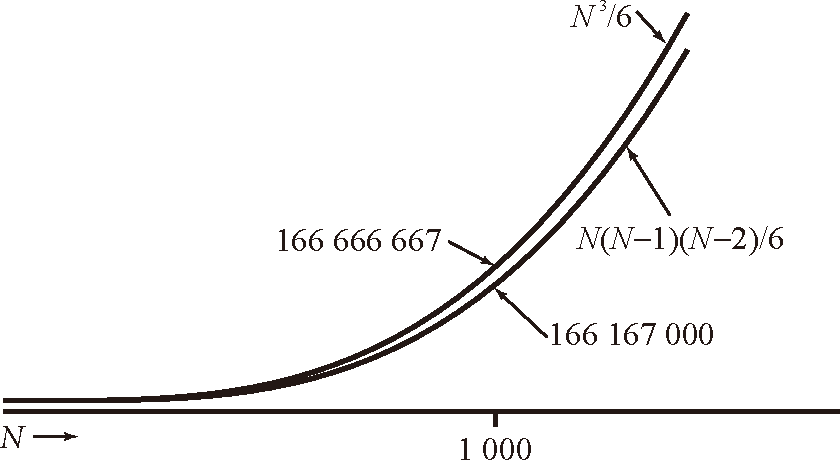# 算法（4th ed）(152)：基础——算法分析 6.3.1(数学模型：近似）

$N(N-1)(N-2)/6=N^3/6-N^2/2+N/3$$N^3/6-N^2/2+N/3$ $\sim N^3/6$ $N^3$
$N^2/2-N/2$ $\sim N^2/2$ $N^2$
$\lg N+1$ $\sim\lg N$ $\lg N$
3 $\sim3$ 1